# CM7-0_ Center of mass.pdf

26 de May de 2023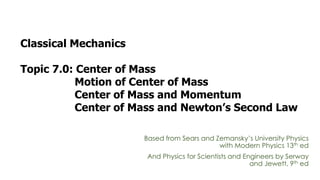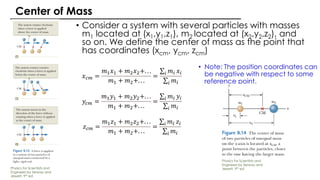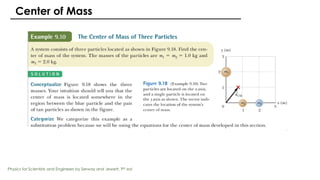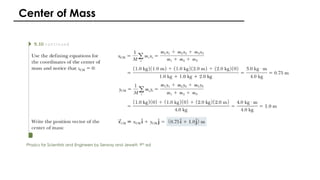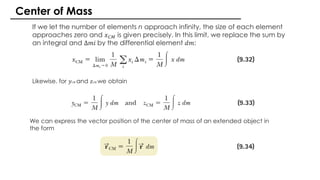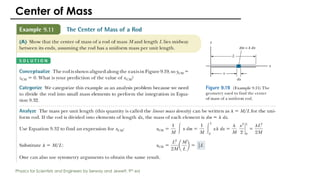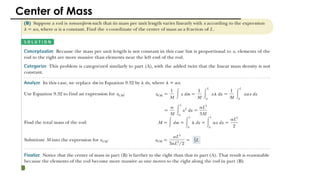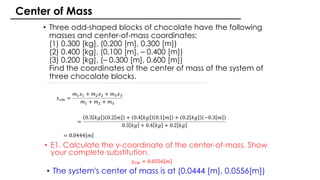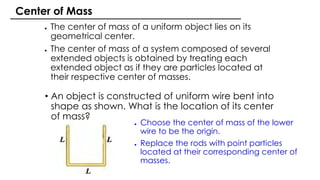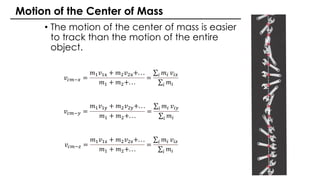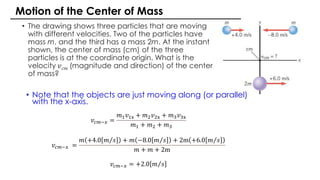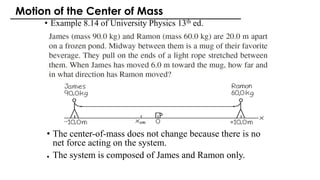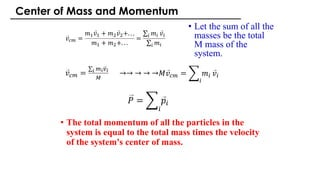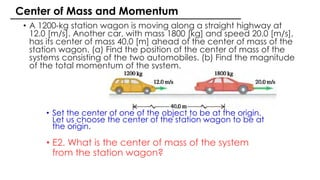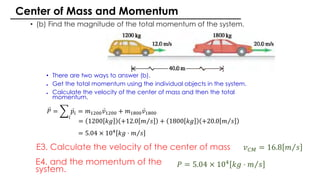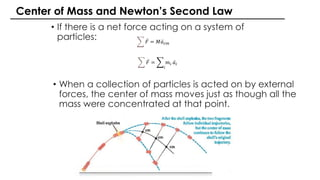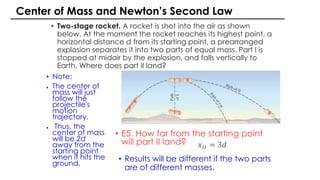1 de 17

### CM7-0_ Center of mass.pdf

• 1. Classical Mechanics Topic 7.0: Center of Mass Motion of Center of Mass Center of Mass and Momentum Center of Mass and Newton’s Second Law Based from Sears and Zemansky’s University Physics with Modern Physics 13th ed And Physics for Scientists and Engineers by Serway and Jewett, 9th ed
• 2. • Consider a system with several particles with masses m1 located at (x1,y1,z1), m2 located at (x2,y2,z2), and so on. We define the center of mass as the point that has coordinates (xcm, ycm, zcm) • Note: The position coordinates can be negative with respect to some reference point. Center of Mass 𝑥𝑐𝑚 = 𝑚1𝑥1 + 𝑚2𝑥2+. . . 𝑚1 + 𝑚2+. . . = σ𝑖 𝑚𝑖 𝑥𝑖 σ𝑖 𝑚𝑖 𝑦𝑐𝑚 = 𝑚1𝑦1 + 𝑚2𝑦2+. . . 𝑚1 + 𝑚2+. . . = σ𝑖 𝑚𝑖 𝑦𝑖 σ𝑖 𝑚𝑖 𝑧𝑐𝑚 = 𝑚1𝑧1 + 𝑚2𝑧2+. . . 𝑚1 + 𝑚2+. . . = σ𝑖 𝑚𝑖 𝑧𝑖 σ𝑖 𝑚𝑖 Physics for Scientists and Engineers by Serway and Jewett, 9th ed Physics for Scientists and Engineers by Serway and Jewett, 9th ed
• 3. Center of Mass Physics for Scientists and Engineers by Serway and Jewett, 9th ed
• 4. Center of Mass Physics for Scientists and Engineers by Serway and Jewett, 9th ed
• 5. If we let the number of elements n approach infinity, the size of each element approaches zero and 𝑥𝐶𝑀 is given precisely. In this limit, we replace the sum by an integral and Δ𝑚𝑖 by the differential element 𝑑𝑚: Likewise, for 𝑦𝐶𝑀 and 𝑧𝐶𝑀 we obtain Center of Mass We can express the vector position of the center of mass of an extended object in the form
• 6. Center of Mass Physics for Scientists and Engineers by Serway and Jewett, 9th ed
• 8. 𝑥𝑐𝑚 = 𝑚1𝑥1 + 𝑚2𝑥2 + 𝑚3𝑥3 𝑚1 + 𝑚2 + 𝑚3 = 0.3 𝑘𝑔 0.2 𝑚 + 0.4 𝑘𝑔 0.1 𝑚 + 0.2 𝑘𝑔 −0.3 𝑚 0.3 𝑘𝑔 + 0.4 𝑘𝑔 + 0.2 𝑘𝑔 = 0.0444 𝑚 • Three odd-shaped blocks of chocolate have the following masses and center-of-mass coordinates: (1) 0.300 [kg], (0.200 [m], 0.300 [m]) (2) 0.400 [kg], (0.100 [m], – 0.400 [m]) (3) 0.200 [kg], (– 0.300 [m], 0.600 [m]) Find the coordinates of the center of mass of the system of three chocolate blocks. • The system's center of mass is at (0.0444 [m], 0.0556[m]) • E1. Calculate the y-coordinate of the center-of-mass. Show your complete substitution. 𝑦𝐶𝑀 = 0.0556 𝑚 Center of Mass
• 9. ● The center of mass of a uniform object lies on its geometrical center. ● The center of mass of a system composed of several extended objects is obtained by treating each extended object as if they are particles located at their respective center of masses. • An object is constructed of uniform wire bent into shape as shown. What is the location of its center of mass? ● Choose the center of mass of the lower wire to be the origin. ● Replace the rods with point particles located at their corresponding center of masses. Center of Mass
• 10. 𝑣𝑐𝑚−𝑥 = 𝑚1𝑣1x + 𝑚2𝑣2x+. . . 𝑚1 + 𝑚2+. . . = σ𝑖 𝑚𝑖 𝑣𝑖𝑥 σ𝑖 𝑚𝑖 𝑣𝑐𝑚−𝑦 = 𝑚1𝑣1y + 𝑚2𝑣2y+. . . 𝑚1 + 𝑚2+. . . = σ𝑖 𝑚𝑖 𝑣𝑖𝑦 σ𝑖 𝑚𝑖 𝑣𝑐𝑚−𝑧 = 𝑚1𝑣1z + 𝑚2𝑣2z+. . . 𝑚1 + 𝑚2+. . . = σ𝑖 𝑚𝑖 𝑣𝑖𝑧 σ𝑖 𝑚𝑖 • The motion of the center of mass is easier to track than the motion of the entire object. Motion of the Center of Mass
• 11. • The drawing shows three particles that are moving with different velocities. Two of the particles have mass m, and the third has a mass 2m. At the instant shown, the center of mass (cm) of the three particles is at the coordinate origin. What is the velocity 𝑣𝑐𝑚 (magnitude and direction) of the center of mass? • Note that the objects are just moving along (or parallel) with the x-axis. Motion of the Center of Mass 𝑣𝑐𝑚−𝑥 = 𝑚1𝑣1x + 𝑚2𝑣2x + 𝑚3𝑣3x 𝑚1 + 𝑚2 + 𝑚3 𝑣𝑐𝑚−𝑥 = 𝑚 +4.0 Τ 𝑚 𝑠 + 𝑚 −8.0 Τ 𝑚 𝑠 + 2m +6.0 Τ 𝑚 𝑠 𝑚 + 𝑚 + 2m 𝑣𝑐𝑚−𝑥 = +2.0 Τ 𝑚 𝑠
• 12. • Example 8.14 of University Physics 13th ed. • The center-of-mass does not change because there is no net force acting on the system. ● The system is composed of James and Ramon only. Motion of the Center of Mass
• 13. • The total momentum of all the particles in the system is equal to the total mass times the velocity of the system's center of mass. Ԧ 𝑣𝑐𝑚 = 𝑚1 Ԧ 𝑣1 + 𝑚2 Ԧ 𝑣2+. . . 𝑚1 + 𝑚2+. . . = σ𝑖 𝑚𝑖 Ԧ 𝑣𝑖 σ𝑖 𝑚𝑖 • Let the sum of all the masses be the total M mass of the system. Ԧ 𝑣𝑐𝑚 = σ𝑖 𝑚𝑖𝑣𝑖 𝑀 →→ → → →𝑀 Ԧ 𝑣𝑐𝑚 = ෍ 𝑖 𝑚𝑖 Ԧ 𝑣𝑖 𝑃 = ෍ 𝑖 Ԧ 𝑝𝑖 Center of Mass and Momentum
• 14. • A 1200-kg station wagon is moving along a straight highway at 12.0 [m/s]. Another car, with mass 1800 [kg] and speed 20.0 [m/s], has its center of mass 40.0 [m] ahead of the center of mass of the station wagon. (a) Find the position of the center of mass of the systems consisting of the two automobiles. (b) Find the magnitude of the total momentum of the system. • Set the center of one of the object to be at the origin. Let us choose the center of the station wagon to be at the origin. • E2. What is the center of mass of the system from the station wagon? Center of Mass and Momentum
• 15. • (b) Find the magnitude of the total momentum of the system. • There are two ways to answer (b). ● Get the total momentum using the individual objects in the system. ● Calculate the velocity of the center of mass and then the total momentum. 𝑃 = ෍ 𝑖 Ԧ 𝑝𝑖 = 𝑚1200 Ԧ 𝑣1200 + 𝑚1800 Ԧ 𝑣1800 = 1200 𝑘𝑔 +12.0 Τ 𝑚 𝑠 + 1800 𝑘𝑔 +20.0 Τ 𝑚 𝑠 = 5.04 × 104 𝑘𝑔 ⋅ Τ 𝑚 𝑠 E4. and the momentum of the system. E3. Calculate the velocity of the center of mass 𝑣𝐶𝑀 = 16.8 Τ 𝑚 𝑠 𝑃 = 5.04 × 104 𝑘𝑔 ⋅ Τ 𝑚 𝑠 Center of Mass and Momentum
• 16. • If there is a net force acting on a system of particles: ෍ Ԧ 𝐹 = 𝑀 Ԧ 𝑎𝑐𝑚 ෍ Ԧ 𝐹 = ෍ 𝑖 𝑚𝑖 Ԧ 𝑎𝑖 • When a collection of particles is acted on by external forces, the center of mass moves just as though all the mass were concentrated at that point. Center of Mass and Newton’s Second Law
• 17. • Two-stage rocket. A rocket is shot into the air as shown below. At the moment the rocket reaches its highest point, a horizontal distance d from its starting point, a prearranged explosion separates it into two parts of equal mass. Part I is stopped at midair by the explosion, and falls vertically to Earth. Where does part II land? • Note: ● The center of mass will just follow the projectile's motion trajectory. ● Thus, the center of mass will be 2d away from the starting point when it hits the ground. • E5. How far from the starting point will part II land? • Results will be different if the two parts are of different masses. 𝑥𝐼𝐼 = 3𝑑 Center of Mass and Newton’s Second Law